Anúncio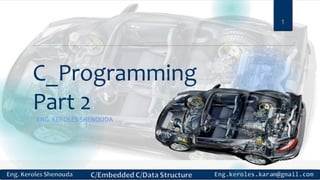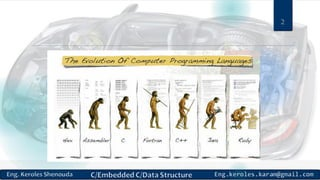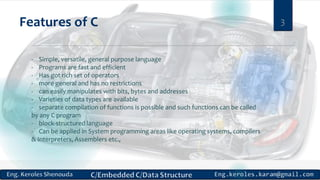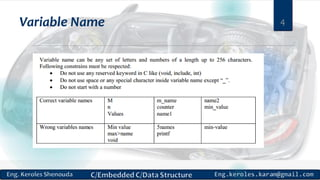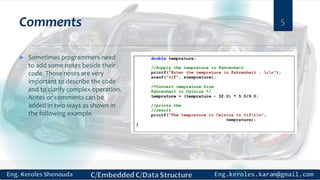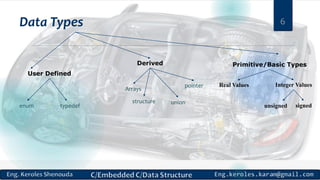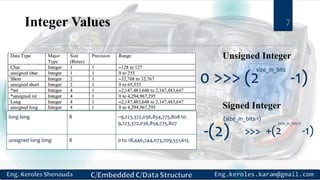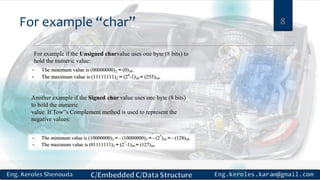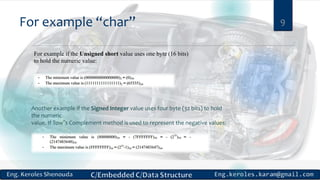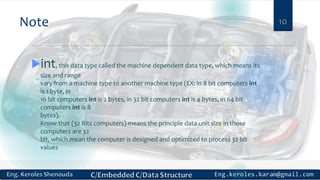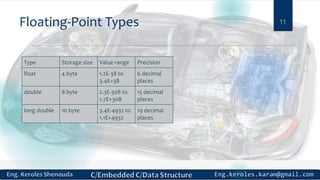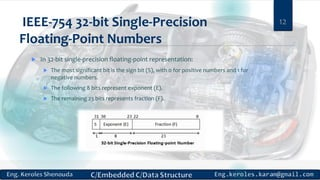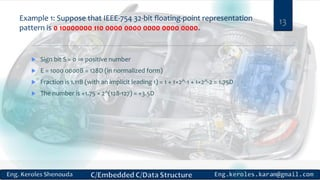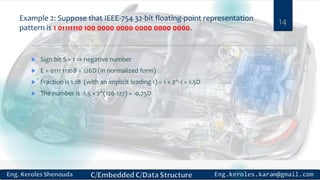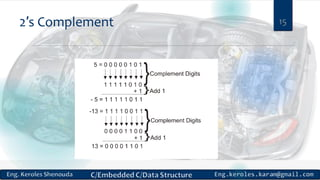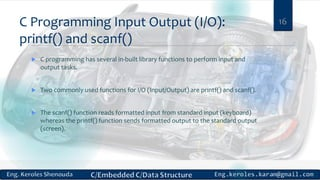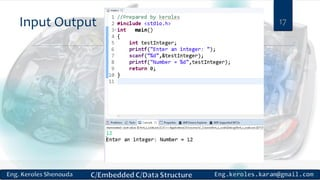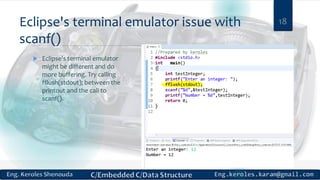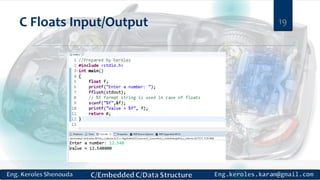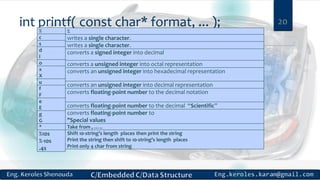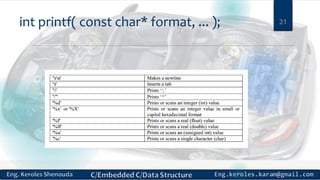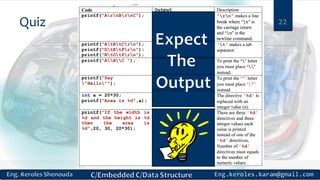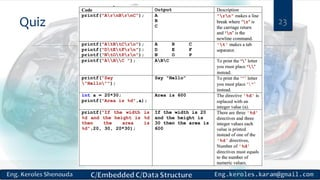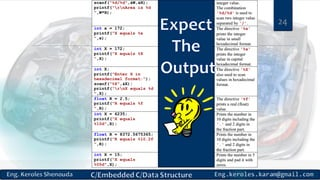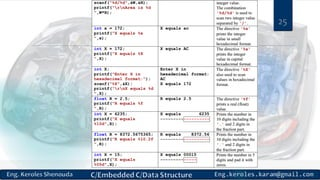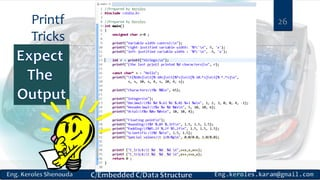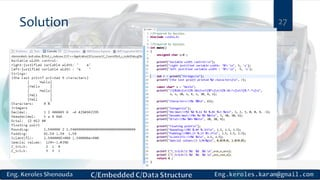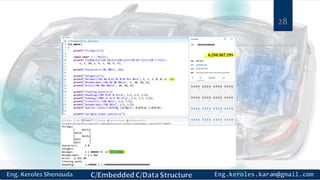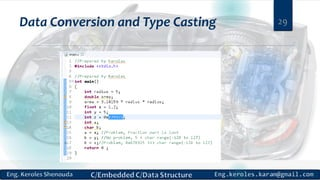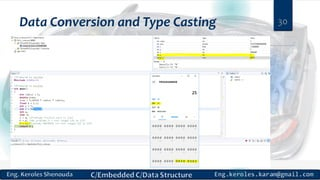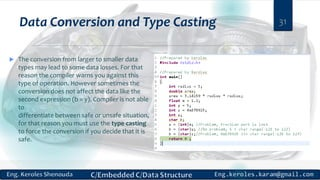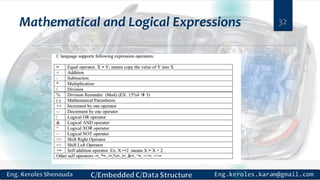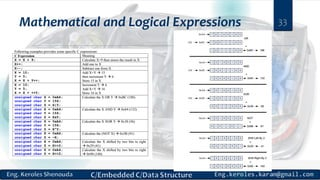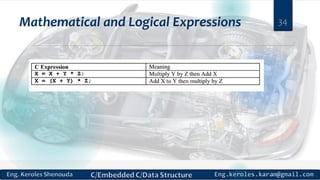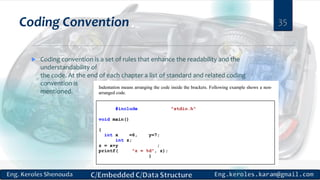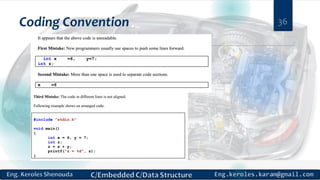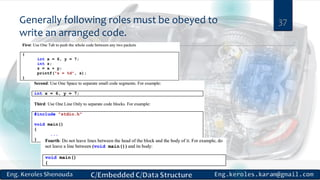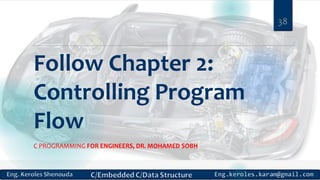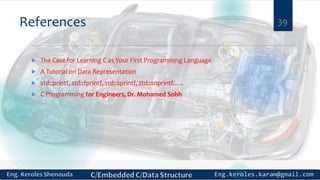1 de 39
Anúncio

### C programming part2

1. C_Programming Part 2 ENG. KEROLES SHENOUDA 1
2. 2
3. Features of C - Simple, versatile, general purpose language - Programs are fast and efficient - Has got rich set of operators - more general and has no restrictions - can easily manipulates with bits, bytes and addresses - Varieties of data types are available - separate compilation of functions is possible and such functions can be called by any C program - block-structured language - Can be applied in System programming areas like operating systems, compilers & Interpreters, Assemblers etc., 3
4. Variable Name 4
5. Comments  Sometimes programmers need to add some notes beside their code. Those notes are very important to describe the code and to clarify complex operation. Notes or comments can be added in two ways as shown in the following example. 5
6. Data Types 6 Primitive/Basic TypesDerived User Defined enum typedef Arrays structure union pointer Integer ValuesReal Values signedunsigned
7. Integer Values 7 long long 8 –9,223,372,036,854,775,808 to 9,223,372,036,854,775,807 unsigned long long 8 0 to 18,446,744,073,709,551,615 Unsigned Integer 0 >>> (2 -1) size_in_bits Signed Integer -(2) (size_in_bits-1) >>> +(2 -1) (size_in_bits-1)
8. For example “char” 8 For example if the Unsigned charvalue uses one byte (8 bits) to hold the numeric value: Another example if the Signed char value uses one byte (8 bits) to hold the numeric value. If Tow‟s Complement method is used to represent the negative values:
9. For example “char” 9 For example if the Unsigned short value uses one byte (16 bits) to hold the numeric value: Another example if the Signed Integer value uses four byte (32 bits) to hold the numeric value. If Tow‟s Complement method is used to represent the negative values:
10. Note int, this data type called the machine dependent data type, which means its size and range vary from a machine type to another machine type (EX: in 8 bit computers int is 1 byte, in 16 bit computers int is 2 bytes, in 32 bit computers int is 4 bytes, in 64 bit computers int is 8 bytes). Know that (32 Bits computers) means the principle data unit size in those computers are 32 bit, which mean the computer is designed and optimized to process 32 bit values 10
11. Floating-Point Types Type Storage size Value range Precision float 4 byte 1.2E-38 to 3.4E+38 6 decimal places double 8 byte 2.3E-308 to 1.7E+308 15 decimal places long double 10 byte 3.4E-4932 to 1.1E+4932 19 decimal places 11
12. IEEE-754 32-bit Single-Precision Floating-Point Numbers  In 32-bit single-precision floating-point representation:  The most significant bit is the sign bit (S), with 0 for positive numbers and 1 for negative numbers.  The following 8 bits represent exponent (E).  The remaining 23 bits represents fraction (F). 12
13. Example 1: Suppose that IEEE-754 32-bit floating-point representation pattern is 0 10000000 110 0000 0000 0000 0000 0000.  Sign bit S = 0 ⇒ positive number  E = 1000 0000B = 128D (in normalized form)  Fraction is 1.11B (with an implicit leading 1) = 1 + 1×2^-1 + 1×2^-2 = 1.75D  The number is +1.75 × 2^(128-127) = +3.5D 13
14. Example 2: Suppose that IEEE-754 32-bit floating-point representation pattern is 1 01111110 100 0000 0000 0000 0000 0000.  Sign bit S = 1 ⇒ negative number  E = 0111 1110B = 126D (in normalized form)  Fraction is 1.1B (with an implicit leading 1) = 1 + 2^-1 = 1.5D  The number is -1.5 × 2^(126-127) = -0.75D 14
15. 2′s Complement 15
16. C Programming Input Output (I/O): printf() and scanf()  C programming has several in-built library functions to perform input and output tasks.  Two commonly used functions for I/O (Input/Output) are printf() and scanf().  The scanf() function reads formatted input from standard input (keyboard) whereas the printf() function sends formatted output to the standard output (screen). 16
17. Input Output 17
18. Eclipse's terminal emulator issue with scanf()  Eclipse's terminal emulator might be different and do more buffering. Try calling fflush(stdout); between the printout and the call to scanf(). 18
19. C Floats Input/Output 19
20. int printf( const char* format, ... );% c s d i o x X u f F e E g G * %10s %-10s .4s 20 % writes a single character. writes a single character. converts a signed integer into decimal converts a unsigned integer into octal representation converts an unsigned integer into hexadecimal representation converts an unsigned integer into decimal representation converts floating-point number to the decimal notation converts floating-point number to the decimal “Scientific” converts floating-point number to "Special values Take from , ….. Shift 10-string’s length places then print the string Print the string then shift to 10-string’s length places Print only 4 char from string
21. int printf( const char* format, ... ); 21
22. Quiz 22
23. Quiz 23
24. 24
25. 25
26. Printf Tricks 26
27. Solution 27
28. 28
29. Data Conversion and Type Casting 29
30. Data Conversion and Type Casting 30
31. Data Conversion and Type Casting 31  The conversion from larger to smaller data types may lead to some data losses. For that reason the compiler warns you against this type of operation. However sometimes the conversion does not affect the data like the second expression (b = y). Compiler is not able to differentiate between safe or unsafe situation, for that reason you must use the type casting to force the conversion if you decide that it is safe.
32. Mathematical and Logical Expressions 32
33. Mathematical and Logical Expressions 33
34. Mathematical and Logical Expressions 34
35. Coding Convention  Coding convention is a set of rules that enhance the readability and the understandability of the code. At the end of each chapter a list of standard and related coding convention is mentioned. 35
36. Coding Convention 36
37. Generally following roles must be obeyed to write an arranged code. 37
38. Follow Chapter 2: Controlling Program Flow C PROGRAMMING FOR ENGINEERS, DR. MOHAMED SOBH 38
39. References 39  The Case for Learning C as Your First Programming Language  A Tutorial on Data Representation  std::printf, std::fprintf, std::sprintf, std::snprintf…..  C Programming for Engineers, Dr. Mohamed Sobh
Anúncio